## 17 January 2014

Congruence of Plane Figures• If two objects are of exactly the same shape and size, they are said to be congruent and the relation between the two objects being congruent is called congruence.
• The method of superposition examines the congruence of plane figures, line segments and angles.
• A plane figure is any shape that can be drawn in two dimensions.
• Two plane figures are congruent, if each, when superimposed on the other, covers it exactly.
• If two line segments have the same or equal length, they are congruent. Also, if two line segments are congruent, they have the same length.
• If two angles have the same measure, they are congruent. Also, if two angles are congruent, their measures are the same.
• If two angles are congruent, the length of their arms do not matter.

Criteria for Congruence of Triangles

#### Congruence of triangles:

Consider triangles ABC and XYZ. Cut triangle ABC and place it over XYZ. The two triangles cover each other exactly, and they are of the same shape and size. Also notice that A falls on X, B on Y, and C on Z. Also, side AB falls along XY, side BC along YZ, and side AC along XZ. So, we can say that triangle ABC is congruent to triangle XYZ. Symbolically, it is represented as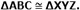So, in general, we can say that two triangles are congruent if all the sides and all the angles of one triangle are equal to the corresponding sides and angles of the other triangle.
In two congruent triangles ABC and XYZ, thecorresponding vertices are A and X, B and Y, and C and Z, that is, A corresponds to X, B to Y, and C to Z. Similarly, the corresponding sides are AB and XY, BC and YZ, and AC and XZ. Also, angle A corresponds to X, B to Y, and C to Z. So, we write ABC corresponds to XYZ.
We can tell if two triangles are congruent using 4 axioms: SAS axiom, ASA axiom, SSS axiom and RHS axiom.
SSS congruence criterion: Two triangles are congruent if three sides of one triangle are equal to the threecorresponding sides of the other triangle.
SAS congruence criterion: Two triangles are congruent if two sides and the included angle of one triangle are equal to the corresponding two sides and the included angle of the other triangle.
RHS congruence criterionTwo right-angled triangles are congruent if the hypotenuse and a side of one triangle are equal to the hypotenuse and the corresponding side of the other triangle.
ASA congruence criterion: Two triangles are congruent if two angles and the included side of one triangle are equal to the corresponding two angles and the included side of the other triangle.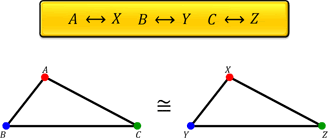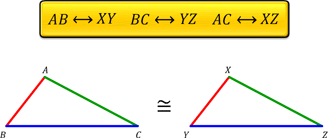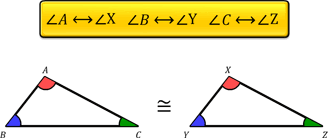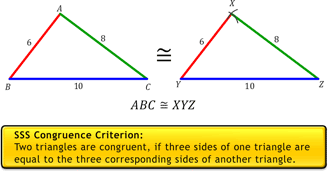We can tell if two triangles are congruent using 4 axioms: SAS axiom, ASA axiom, SSS axiom and RHS axiom.
SSS congruence criterion: Two triangles are congruent if three sides of one triangle are equal to the threecorresponding sides of the other triangle.
SAS congruence criterion: Two triangles are congruent if two sides and the included angle of one triangle are equal to the corresponding two sides and the included angle of the other triangle.
RHS congruence criterionTwo right-angled triangles are congruent if the hypotenuse and a side of one triangle are equal to the hypotenuse and the corresponding side of the other triangle.
ASA congruence criterion: Two triangles are congruent if two angles and the included side of one triangle are equal to the corresponding two angles and the included side of the other triangle.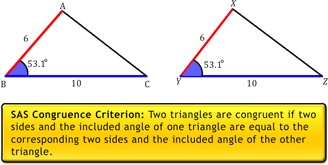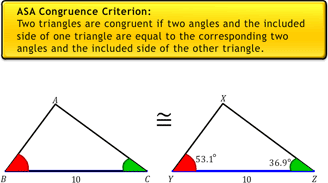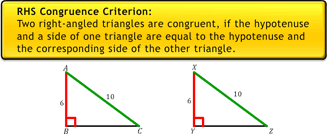With our free PDF notes you can get success in any competitive or entrance exams like CTET,  KVS, NET, CAT, MAT, CMAT, SSC, B.ED, IBPS Recruitment, IAS, CSAT, State Civil Services Exams, UPTET, PSTET, HTET & many more. It also provides NCERT solutions, CBSE, NTSE, Olympiad study material, Indian General Knowledge, English, Hindi, Mathematics, Current affairs, Science, S.ST, model test papers, important Questions and Answers asked in CBSE examinations.